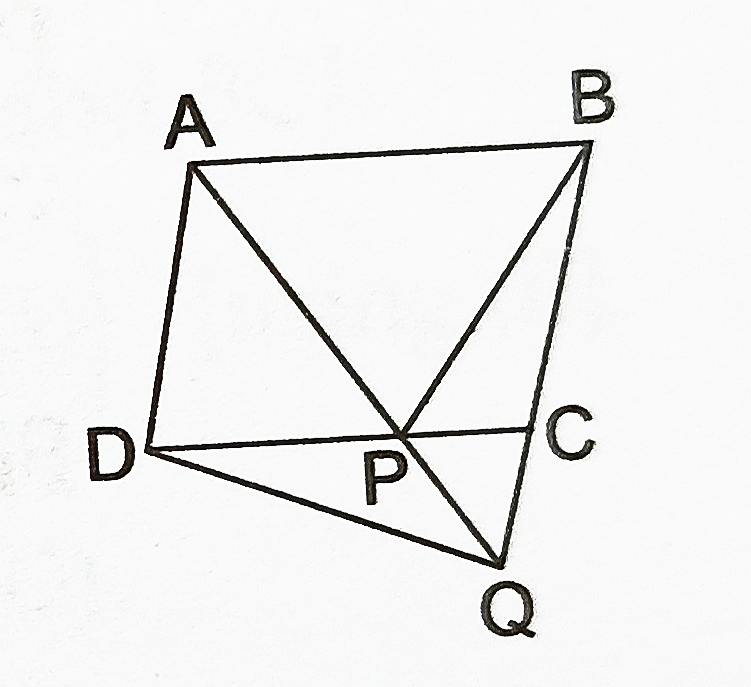# Class 9 Areas of Parallelograms and Triangles Important Questions

Areas of Parallelograms and Triangles Important Questions

1. If AD is one of the medians of a triangle and P is a point on AD. Prove that
1. ar(∆BDP) = ar(∆CDP)
2. ar(∆ABP) = ar(∆ACP)
2. In the adjoining figure, D is the mid-point of side AB of ∆ABC and P is any point on BC. If CQ||PD meets AB in Q, prove that ar(∆BPQ) = 1/2 ar(∆ABC).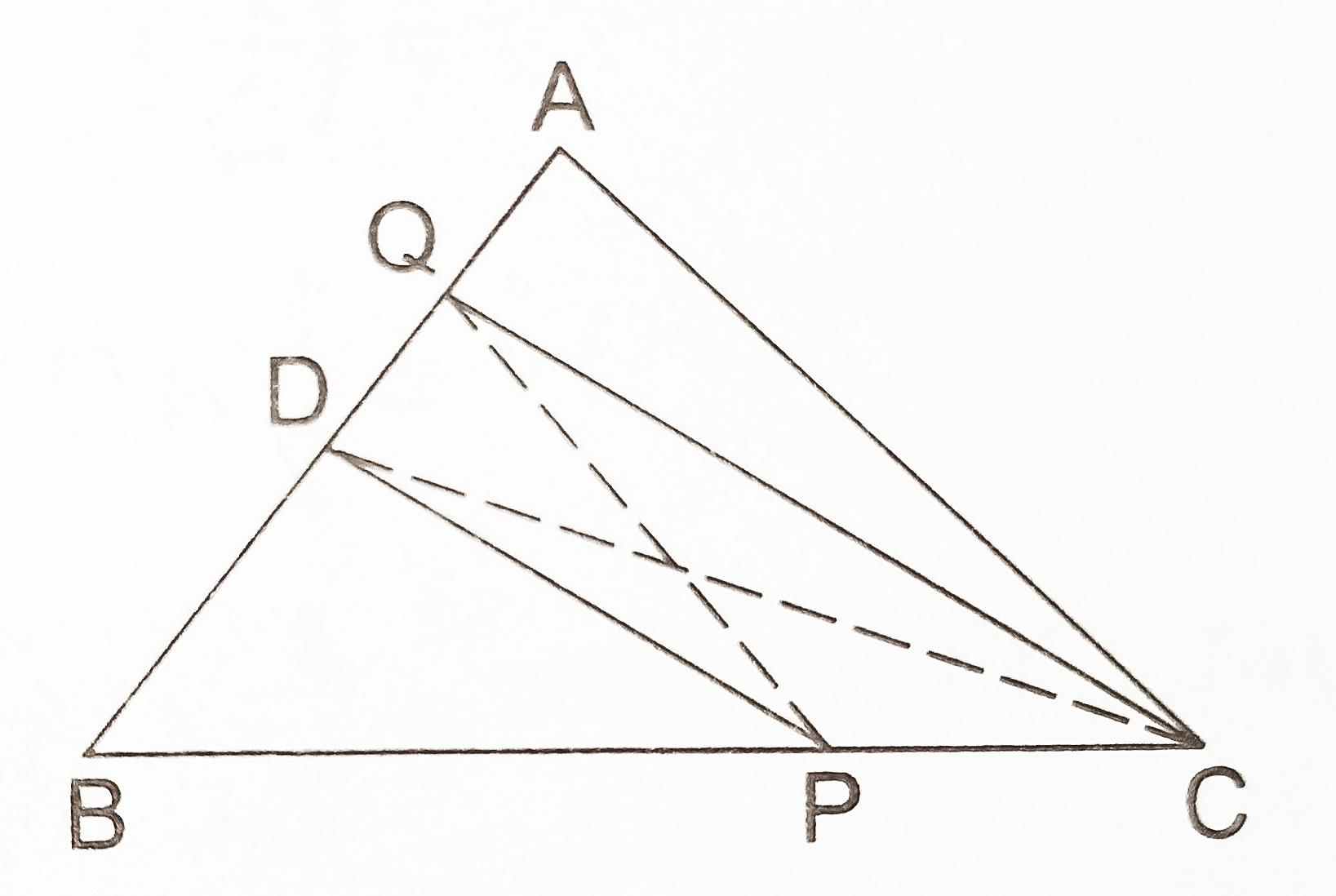3. A point O inside a rectangle ABCD is joined to the vertices. Prove that the sum of the areas of a pair of opposite triangles so formed is equal to the sum of the areas of the other pair of triangles.
4. D, E, F are the midpoints of the sides BC, CA and AB respectively of ∆ ABC. Prove that
1. BDEF is a parallelogram,
2. ar(∆DEF) = 1/4 ar(∆ABC) and
3. ar(||gm BDEF) =1/2ar(∆ABC).
5. In the adjoining figure, PQRS and PABC are two parallelograms of equal area. Prove that QC||BR.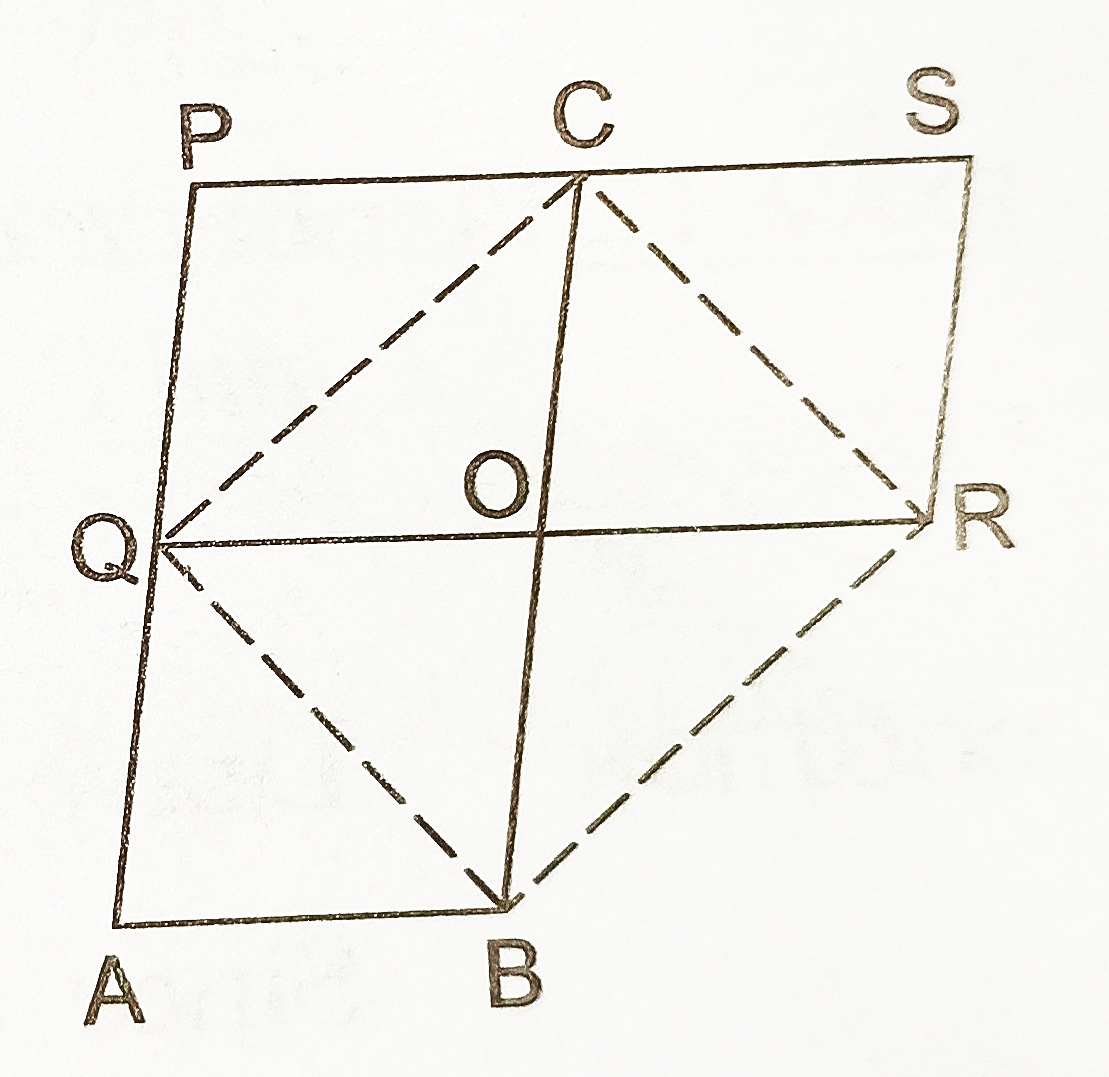6. X and Y are points on the side LN of the triangle LMN, such that LX = XY = YN. Through X, a line is drawn parallel to LM to meet MN at Z(see figure). Prove that ar(LZY) = ar (quad. MZYX).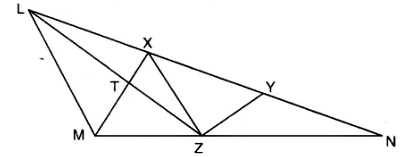7. ABCD is a parallelogram in which BC is produced to E such the CE = BC. AE intersects CD at F (see figure). IF area of DFB = 3 cm2, find the area of the parallelogram ABCD.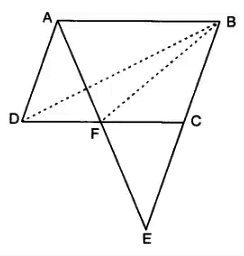8. ABCD is a parallelogram and P is any point in its interior. Show that ar(APB) + ar (CPD) = ar(BPC) + ar(APD).
9. ABCD is a parallelogram and BC is produced to a point Q such that AD= CQ. If AQ intersects DC at P, show that area of ΔBPC= area of ΔDPQ.
10. ABCD is a trapezium in which AB is parallel to DC, DC = 40 cm and AB = 60 cm. If X and Y are the mid – points of AD and BC respectively, prove that:
1. XY = 50 cm
2. DCYX is a trapezium
3. ar (trap. DCYX) = (9/11)ar (trap.(XYBA).
11. Let P ,Q ,R ,S be respectively the mid points of the sides AB, BC, CD and DA of quadrilateral ABCD . Show that PQRS is a parallelogram such that ar(parallelogram PQRS) = 1/2 ar(quad. ABCD).
12. The area of parallelogram PQRS is 88 cm2. A perpendicular from S is drawn to intersect PQ at M. If SM = 8 cm, then find the length of PQ.
13. ABCD is a parallelogram and O is a point in its interior. Prove that ar (∆AOB) + ar(COD) = 1/2 ar (parallelogram ABCD).
14. In Figure, diagonals AC and BD of quadrilateral ABCD intersect at O such that OB = OD. If AB = CD, then show that:
1. ar (DOC) = ar (AOB)
2. ar (DCB) = ar (ACB)
3. DA || CB or ABCD is a parallelogram.15. In the adjoining figure, ABCD is a parallelogram and a line through A cuts DC at P and BC produced at Q. Prove that ar(BPC) = ar(DPQ).